# 间接解密

## Windows平台

### 方法剖析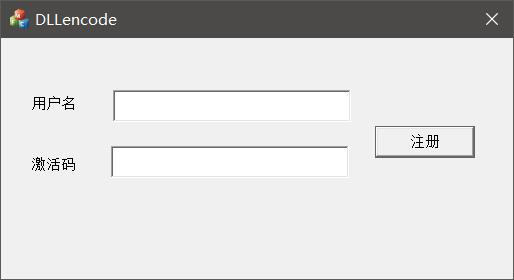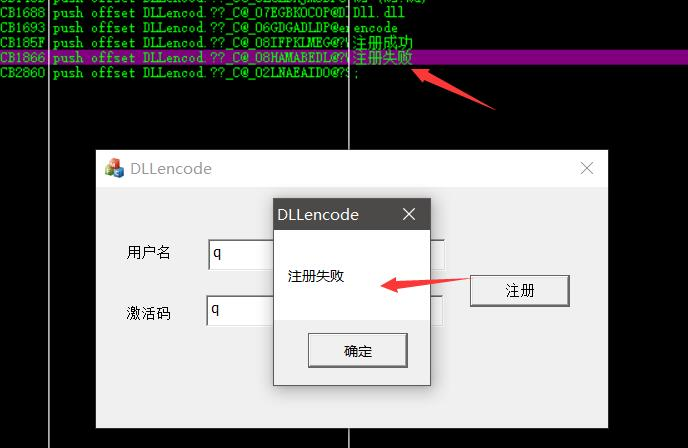``````0FEF103E  |.  5B            pop ebx                                               ;  DLLencod.00CB1827
0FEF103F  |>  5F            pop edi                                               ;  DLLencod.00CB1827
0FEF1040  |.  C60430 00     mov byte ptr ds:[eax+esi],0x0
0FEF1044  |.  5E            pop esi                                               ;  DLLencod.00CB1827
0FEF1045  |.  5D            pop ebp                                               ;  DLLencod.00CB1827
0FEF1046  \.  C3            retn

``````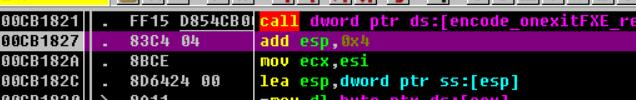``````typedef  char*(__cdecl *lpencodefun)(char*);
``````

### 注册机编写

``````#include "stdafx.h"
#include <Windows.h>

int _tmain(int argc, _TCHAR* argv[])
{

typedef  char*(__cdecl *lpencodefun)(char*);

printf("%x\n",h+0x1000);

lpencodefun encode = (lpencodefun)(h+0x1000);
printf("%s\n",encode("abc"));
system("pause");
return 0;
}
``````

## Android偷取加密算法

### 抓包

``````as=ab9aacee485e79df9e9aac&mas=01999323139999f9b9b9a379b945a1c446f9b9b9a399a35919a3d3
``````

java层的分析还是相对而言简单一些的，所以我们往上看

``````v15_2 = v6.a();
v0_5 = AppLog.l();
String v14_2 = !m.a(v0_5) ? UserInfo.getUserInfo(v5_1, v15_2, v14_1, v0_5) : UserInfo.getUserInfo(v5_1, v15_2, v14_1, "");
if(!TextUtils.isEmpty(((CharSequence)v14_2))) {
int v15_3 = v14_2.length();
if(v15_3 % 2 == 0) {
v14_2 = v14_2.substring(0, v15_3 >> 1);
this.h = StcSDKFactory.getSDK(this.d, this.c);
this.h.SetRegionType(0);
if(!this.e) {
v15_2 = AppLog.h();
if(!TextUtils.isEmpty(((CharSequence)v0_5)) && !TextUtils.isEmpty(((CharSequence)v15_2))) {
this.h.setParams(v0_5, v15_2);
this.e = true;
}
}

this.h.setSession(a.a());
v15_2 = d.a(this.h.encode(v14_2.getBytes()));
}
else {
v14_2 = "a1qwert123";
v15_2 = "123";
}
}
else {
v14_2 = "a1iosdfgh";
v15_2 = "123";
}

v6.a("as", v14_2);
v6.a("mas", v15_2);
``````

``````v15_2 = d.a(this.h.encode(v14_2.getBytes()));
``````

mas是通过d.a这个方法进行计算的，虽然混淆很严重，但是分析起来还不是很烦，跟进这个a方法。

``````static {
d.a = new char[]{'0', '1', '2', '3', '4', '5', '6', '7', '8', '9', 'a', 'b', 'c', 'd', 'e', 'f'};
}
public static String a(byte[] arg2) {
if(arg2 == null) {
throw new NullPointerException("bytes is null");
}

return d.a(arg2, 0, arg2.length);
}
``````

``````public static String a(byte[] arg9, int arg10, int arg11) {
if(arg9 == null) {
throw new NullPointerException("bytes is null");
}

if(arg10 >= 0) {
if(arg10 + arg11 > arg9.length) {
}
else {
int v0 = arg11 * 2;
char[] v1 = new char[v0];
int v3 = 0;
int v4 = 0;
while(v3 < arg11) {
int v5 = arg9[v3 + arg10] & 255;
int v6 = v4 + 1;
v1[v4] = d.a[v5 >> 4];
v4 = v6 + 1;
v1[v6] = d.a[v5 & 15];
++v3;
}

return new String(v1, 0, v0);
}
}

throw new IndexOutOfBoundsException();
}
``````

``````this.h.encode(v14_2.getBytes())
``````

``````private ISdk h;
``````

``````static {
try {
return;
}
return;
}
}
``````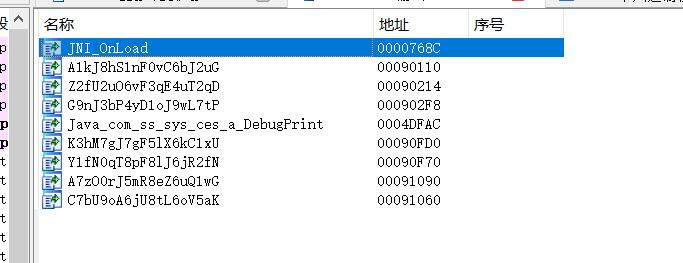### 用魔法打败魔法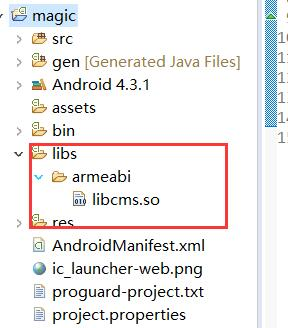``````art/runtime/java_vm_ext.cc:410] JNI DETECTED ERROR IN APPLICATION: JNI NewStringUTF called with pending exception java.lang.ClassNotFoundException: Didn't find class "com.ss.android.common.applog.UserInfo" on path: DexPathList[[zip file "/data/app/com.example.magic-1/base.apk"],nativeLibraryDirectories=[/data/app/com.example.magic-1/lib/arm, /data/app/com.example.magic-1/base.apk!/lib/armeabi, /vendor/lib, /system/lib, /system/lib/arm]]
``````

`Didn't find class "com.ss.android.common.applog.UserInfo"`

2 Likes